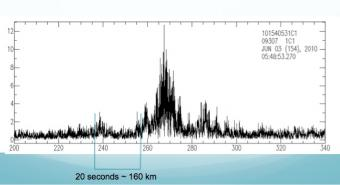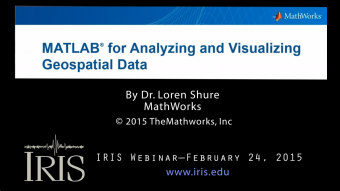## Introduction to Spectral Analysis and Matlab

The object of this lab is to explore the relationship between the time domain and the frequency domain while being introduced to the numerical computing program MATLAB. Students first look at pure sine waves and their superpositions as a function of time and their representation in the frequency domain, and then move on to examine similar features in earthquake data.

This exercise was last updated (5/30/14) by Rob Anthony, Colorado State U. (ranthony3036@gmail.com) and used as part of the IRIS Internship Orientation Week.

#### Objectives:

Students will be able to:

• Understand how to use basic MATLAB functions and plotting commands on data vectors
• Write a multi-cell script in MATLAB
• Have a cursory understanding of how to calculate and interpret seismic spectra
• Read, filter, and compute/plot the seismic spectra of earthquake data from SAC files

## Related LessonsThis tutorial provides student with basic tools and procedures generally used to explore broadband seismic data in Matlab.